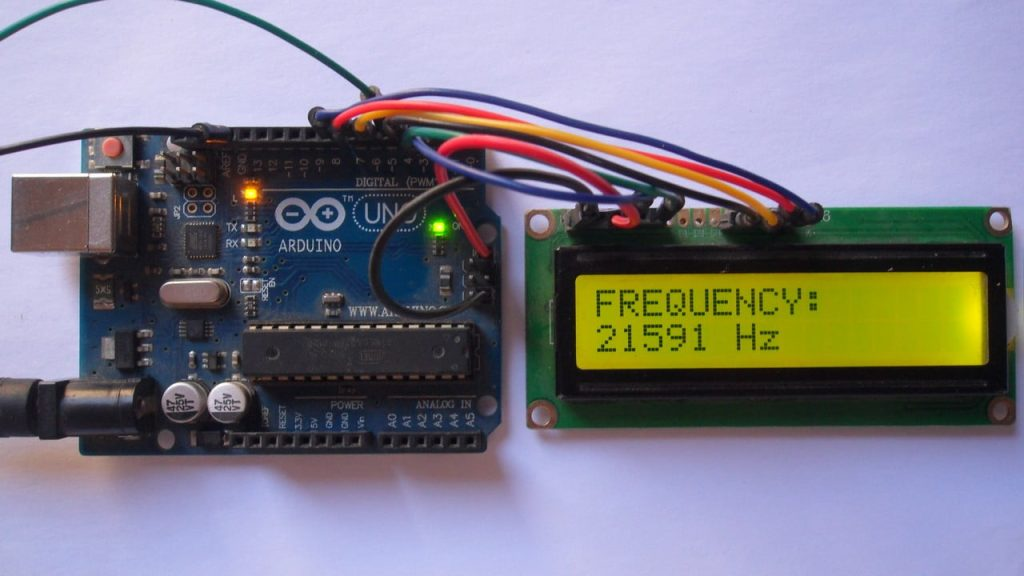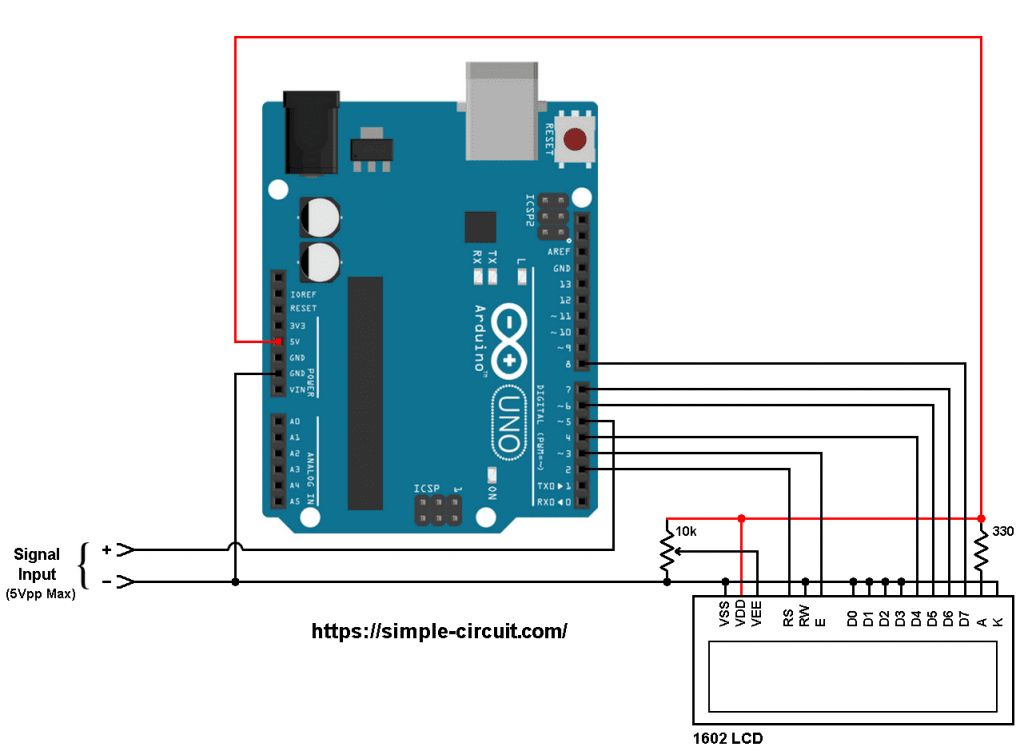# Arduino Frequency Counter | Arduino Projects

This post shows how to build a frequency counter device using Arduino UNO board where signal frequency value is displayed on 1602 LCD screen.
With this counter we can measure frequency of PWM signals with peak voltage of 5V.

Related Project:
220/380V AC Frequency Meter with Arduino

Hardware Required:
This is a list of all components required to build this project.

• Arduino UNO board   —-> Atmega328P datasheet
• 16×2 LCD screen
• 330 ohm resistor
• 10k ohm variable resistor or potentiometer
• Jumper wiresArduino frequency counter circuit:
Project circuit diagram is shown below.

The 16×2 LCD screen (2 rows and 16 columns) is used to display the values of frequency and period of the input voltage where:
RS —> Arduino digital pin 2
E   —> Arduino digital pin 3
D4 —> Arduino digital pin 4
D5 —> Arduino digital pin 6
D6 —> Arduino digital pin 7
D7 —> Arduino digital pin 8
VSS, RW, D0, D1, D2, D3 and K are connected to Arduino GND,
VEE to the 10k ohm variable resistor (or potentiometer) output,
VDD to Arduino 5V and A to Arduino 5V through 330 ohm resistor.

VEE pin is used to control the contrast of the LCD. A (anode) and K (cathode) are the back light LED pins.The PWM signal has two pins, let’s say positive (+) and negative (-), they are connected to the circuit as shown above.
The positive terminal is connected to Arduino digital pin 5 and the negative terminal is connected to Arduino GND pin.

Arduino frequency counter code
The following Arduino code requires a library which helps doing this project easily. This library is named FreqCount, it can be installed online through Arduino library manger (Manage Libraries…) or manually by downloading and installing its zip file. Download link is below:
Arduino FreqCount library    —-> direct link

The FreqCount library uses Timer/Counter1 module to count number of pulses during fixed time, Timer/Counter2 module is used for this fixed time.

Full Arduino code:

The video below shows a protoboard circuit of the project:

This site uses Akismet to reduce spam. Learn how your comment data is processed.